Ch 1. Particle General Motion Multimedia Engineering Dynamics Position,Vel & Accel. Accel. varyw/ Time Accel. Constant Rect. Coordinates Norm/Tang. Coordinates Polar Coordinates RelativeMotion
 Chapter - Particle - 1. General Motion 2. Force & Accel. 3. Energy 4. Momentum - Rigid Body - 5. General Motion 6. Force & Accel. 7. Energy 8. Momentum 9. 3-D Motion 10. Vibrations Appendix Basic Math Units Basic Equations Sections Search eBooks Dynamics Fluids Math Mechanics Statics Thermodynamics Author(s): Kurt Gramoll ©Kurt GramollDYNAMICS - CASE STUDY SOLUTION Velocity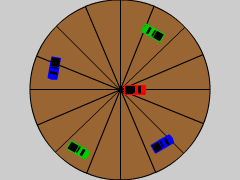General Motion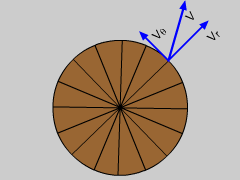Velocity Motion The velocity of the truck can be determined using velocity equation in polar coordinates,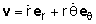where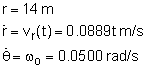v = 0.0889t er + 14(0.0500) eθ However, this is still a function of t. To find the time time t required for the truck to travel from r = 4 to r = 14 meters, integrate the radial component of the velocity, giving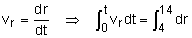(0.0889 t2)/2 = 14 - 4      t = 15 s Now, substitute t into the equation to calculate the final velocity, v,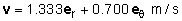Acceleration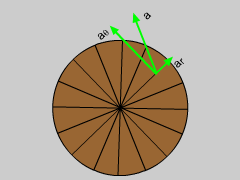Acceleration Motion Similarly, the acceleration equation can be used to calculate the acceleration of the truck when it is 14 meters from the center of the carousel. Since the time has already be determined previously, no integration is required.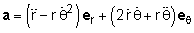where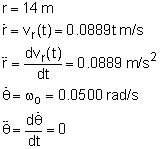a = [0.0889 - 14(0.0500)2]er                            + [2(0.0889)(15)(0.0500) - 0]eθ      a = 0.5390er + 0.1334eθ

Practice Homework and Test problems now available in the 'Eng Dynamics' mobile app
Includes over 400 problems with complete detailed solutions.
Available now at the Google Play Store and Apple App Store.